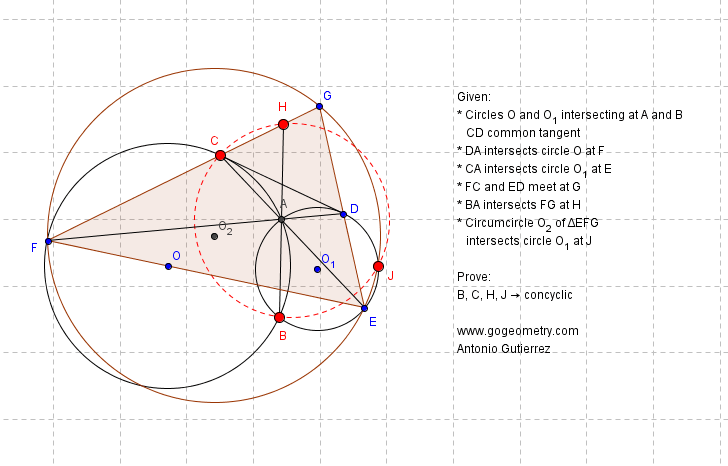###### Static Diagram Geometry Problem 901: Intersecting Circles, Common External Tangent, Secant, Circumcircle, Concyclic Points, Cyclic Quadrilateral. Levels: School, College, Mathematics Education

< PREVIOUS PROBLEM  |  NEXT PROBLEM >The circles O and O1 intersect at A and B (see the figure above), CD is the common external tangent, CA intersects circle O1 at E, DA intersects circle O at F, FC and ED intersect at G, BA intersects FG at H. If the circumcircle of triangle EFG intersects circle O1 at J, prove that the points B, C, H, J are concyclic.
See the dynamic diagram of problem 901

Home | SearchGeometry | Problems | All Problems | Open Problems | Visual Index | 10 Problems | Problems Art Gallery Art | 901-910 | Dynamic Geometry | GeoGebra | Circle | Intersecting Circles | Tangent Line | Circumcircle | Concyclic Points | Cyclic Quadrilateral | Email | Solution/Comment | by Antonio Gutierrez